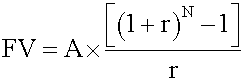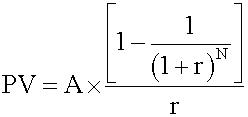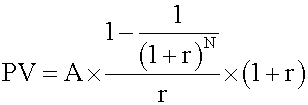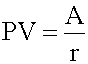### Seeing is believing!

Before you order, simply sign up for a free user account and in seconds you'll be experiencing the best in CFA exam preparation.

##### Subject 2. The Future Value and Present Value of a Series of Equal Cash Flows (Ordinary Annuities, Annuity Dues, and Perpetuities)
Annuity is a finite set of sequential cash flows, all with the same value.

Ordinary annuity has a first cash flow that occurs one period from now (indexed at t = 1). In other words, the payments occur at the end of each period.

• Future value of a regular annuitywhere

• A = annuity amount
• N = number of regular annuity payments
• r = interest rate per period

• Present value of a regular annuityAnnuity due has a first cash flow that is paid immediately (indexed at t = 0). In other words, the payments occur at the beginning of each period.

• Future value of an annuity due

This consists of two parts: the future value of one annuity payment now, and the future value of a regular annuity of (N -1) period. Calculate the two parts and add them together. Alternatively, you can use this formula:Note that, all other factors being equal, the future value of an annuity due is equal to the future value of an ordinary annuity multiplied by (1 + r).

• Present value of an annuity due

This consists of two parts: an annuity payment now and the present value of a regular annuity of (N - 1) period. Use the above formula to calculate the second part and add the two parts together. This process can also be simplified to a formula:Note that, all other factors being equal, the present value of an annuity due is equal to the present value of an ordinary annuity multiplied by (1 + r).

Hint: Remember these formulas - you can use them to solve annuity-related questions directly, or to double-check the answers given by your calculator.

A perpetuity is a perpetual annuity: an ordinary annuity that extends indefinitely. In other words, it is an infinite set of sequential cash flows that have the same value, with the first cash flow occurring one period from now.This equation is valid for a perpetuity with level payments, positive interest rate r. The first payment occurs one period from now (like a regular annuity). An example of a perpetuity is a stock paying constant dividends.

Example: Future value of a regular annuity

An analyst decides to set aside \$10,000 per year in a conservative portfolio projected to earn 8% per annum. If the first payment he makes is one year from now, calculate the accumulated amount at the end of 10 years.

Method 1: Using a formula

• Identify the given variables: A = 10,000, r = 0.08, N = 10
• Identify the appropriate formula: FV = A x {[(1 + r)N - 1] / r}
• Solve for the unknown: FV = 10,000 {[(1 + 0.08)10 - 1] / 0.08} = \$144,865

Method 2: Using a calculator

Texas Instruments settings:

• 2nd P/Y = 1 and key in 1 ENTER.
• SET END since this is a regular annuity. You do this by pressing 2nd BGN 2nd SET until you see END displaying. Press 2nd SET twice if necessary. After setting to END, you must always press 2nd QUIT and then continue.Exploration: Change the problem to an annuity due (i.e., SET BGN) and compare the amounts. (The answer is \$156,454.87 - a difference of \$11,589.25)

User Comment
shakoo excellent
hagi10 do we really need to remmember all these formulas if we are using the calculator?
natthawut I think just use Calculator not remember formular
Gnomez How do I do the PV of an annuity due in the HP12C?
Vernon why are there different answers, hp vs. texas instr. will there be a variance allowed for the answers on the test?
imroark I want to Buy a calculator. Can somebody help me find one?
Win1977 Buy the TI, you can find it at a grand and toy or any office shop, it will say it's appr. for the CFA exam. Good luck.
orchid I set my BA TI to 6 decimal pts., answer is same as hp...I dont think it matters in the exam thou', but in case anyone wonder the difference it can make.
Nathan (1) There's not a difference between the results of the two calculators. (2) Just get the TI-BAII if you don't already have a preference. It's easier to learn, costs less, and reportedly calculates faster, but I'm not sure there's a difference.
Taimoor I also had a difference using TI BA II...my answere comes to 144,865.62
theo with hp 12C:

10000 [chs] pmt
8 i
10 n
fv = 144,865.62
rosh07 good job theo
AndressaS how can i get annuity due of \$156,454.87 with HP?
netty how can one determine the formula to use (Future value ordinary annuity vs future value annuity due) mere looking at a question.whats the major difference one should look out for?
ngeorge For an annuity due on the HP you need to switch to "annuity due mode" by pressing [g] [BEG] (the [BEG] key is the "7" key. You will see the word "BEGIN" on the LCD screen of the calculator after you do this.

It will stay in this mode until you switch back to ordinary annuity mode by pressing [g] [END] ("8" key). Make sure to switch back or you will be in trouble!

FYI, the point of "BEGIN" and "END" is that an annuity due is paid at the BEGINNING of the period and an ordinary annuity is paid at the END of a period.
ngeorge Netty, you should just assume it is an ordinary annuity unless the problem specifically says annuity due or payments are at the beginning of the period.

In the real world, mortgages and most other loans are ordinary annuities and pension annuity payments are the most common type of annuities due.
Ioannis * Don't need to remember all formulas. Rememeber the Geometric series sum formula Sum=A*(1-r^(N+1))/(1-r). Then set A=A the sum, r=r+1 the factor is the interest rate plus 1 and N=N-1 because you start counting from 1 not 0. This comes out as the formula for the FV.

* Then use FV=PV*(1+r)^N to find PV

* Then remember to multiply by (1+r) for annuities dues to account for the one extra period (or set A=A*(1+r), same thing).
youyoumm one correction on the sample set for Texas Instrument,step2 is 2nd CLR TVM,not 2nd CLR RVM,and you won't find this CLR RVM anyway.
jaiaaditya these notes are very useful or easy to understand i am too much happy to find this site and enrolling myself on this
reggie9600 so anyone else getting 144865.625 rather than 144866.62 on the texas calculator? if so any idea why? is this something to do with calculator settings?
TiredHand I get 144865.6247 . . . depends on how detailed you go

Question: How do you use a TI calc for a question such as the PV of an ordinary annuity? Ie. p 274 Quant:

You are considering purchasing a financial asset that promises to pay \$1,000 per year for five years, with the first payment one year from now. The req. rate of return is 12% per year. How much should you pay for this asset?
Rozik Thanks youyoumm! I could not figure out where I am making a mistake. You clarified it for me.
motjoke How do you calculate annuity due on BAII
Ifi2703 To set calculator for Annuity Due on BAII, press:
2nd PMT
2nd ENTER (if it says END, press 2nd ENTER again and it will change to BGN)
Now you can press 2nd CPT (to quit) and you will see BGN in the top right of screen and you know it'll now calculate payments as coming in at the beginning of the period.

Hope this helps!
hussein1978 can i use only formula , without calculator things ,quastion:we can get calculator in exame room??
mbowa Am getting 146,396.12 using the ba11 guess ve done some setting wrong, can anyone help me?
Chl4072 Why I can't get 144865...? I get 156454.87
Question: how do we know when to use annuity formula, when to use normally formula to find FV..?
shamflora1 Hi, I'm getting 146,484.8184 using BAII Plus with a PMT of 10000. If I switch to -10000, the answer is 143,246.4309. Either way not the 144 answer listed. Could anyone help? Thanks in advance.
yMinnie I set the annuity to END and entered -10,000 for PMT, 10 for N, and 8 for I/Y, how do I keep getting the FV of 146,484.8184. Can anyone help? Thanks!!
tgig On BAII, be sure to follow the instructions for clearing memory before doing the calculation. The `2ND QUIT` and `2ND CLR TVM` are important to get the right answer.
zeanww These formulas are got with sufficient amount of knowledge in annuity and perpetuity. Actually the annuity formula above is composed of two perpetuity formulas. I really think AnalystNotes should provide us with more intuition of how these formulas came around, rather than giving out the formula straight away. This is not going to be so beneficial.